# RS Aggarwal Solutions for Class 8 Maths Chapter 12 - Direct and Inverse Proportions

RS Aggarwal Solutions for Class 8 Maths Chapter 12- Direct and Inverse Proportions, are provided here. Our expert faculty team has prepared solutions in order to help you with your exam preparation to acquire good marks in Maths. If you wish to secure an excellent score, solving RS Aggarwal Solutions for Class 8 is an utmost necessity. These solutions will help you in gaining knowledge and strong command over the subject. Practising the textbook questions will help you in analyzing your level of preparation and knowledge of the concept.

In order to help you understand and solve the problems, we at BYJU’S have designed these solutions in a very lucid and clear manner that helps students solve problems in the most efficient possible ways. Here is the RS Aggarwal Solutions for Class 8 Chapter 12 where solutions are solved in detail. Download pdf of Class 8 Chapter 12 in their respective links.

## Download PDF of RS Aggarwal Solutions for Class 8 Maths Chapter 12 – Direct and Inverse Proportions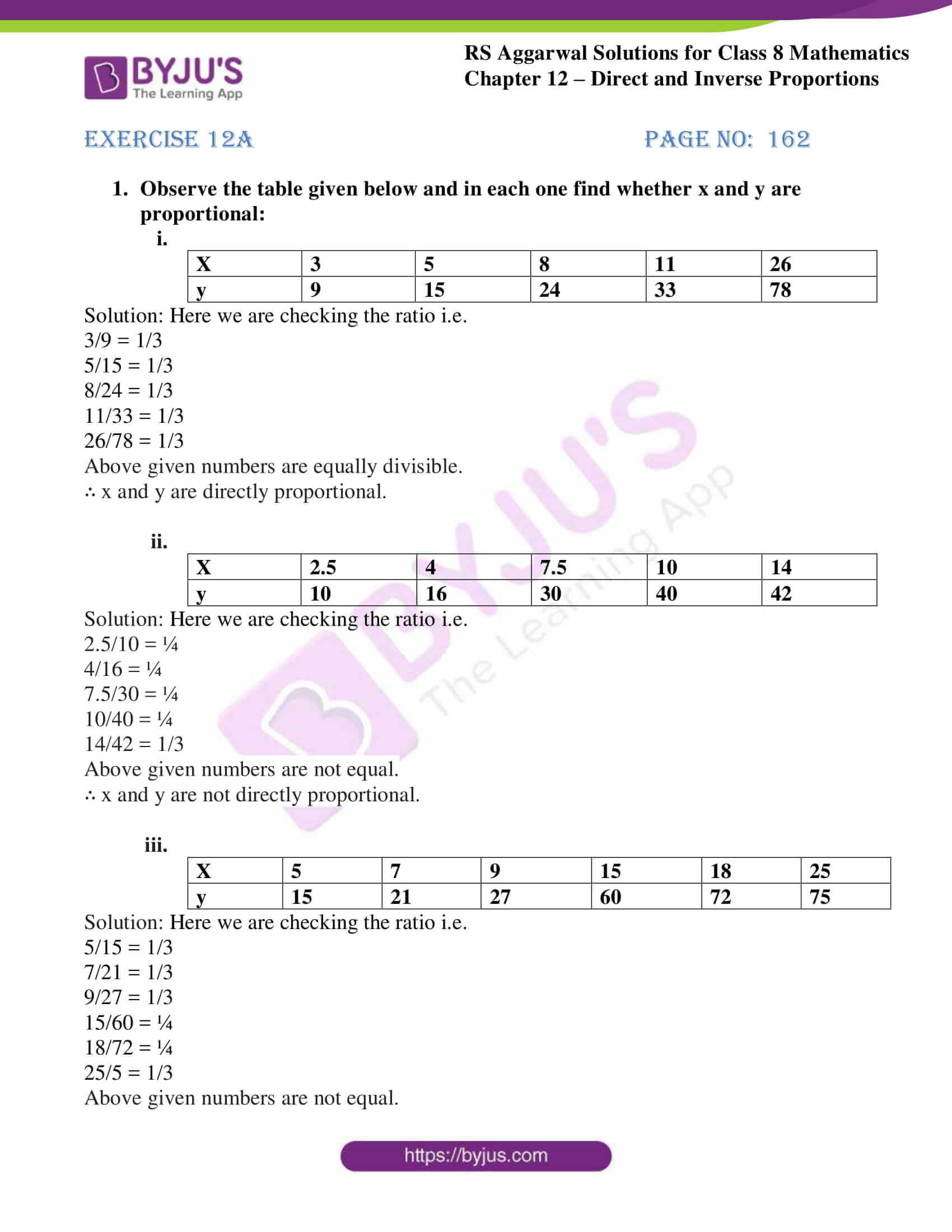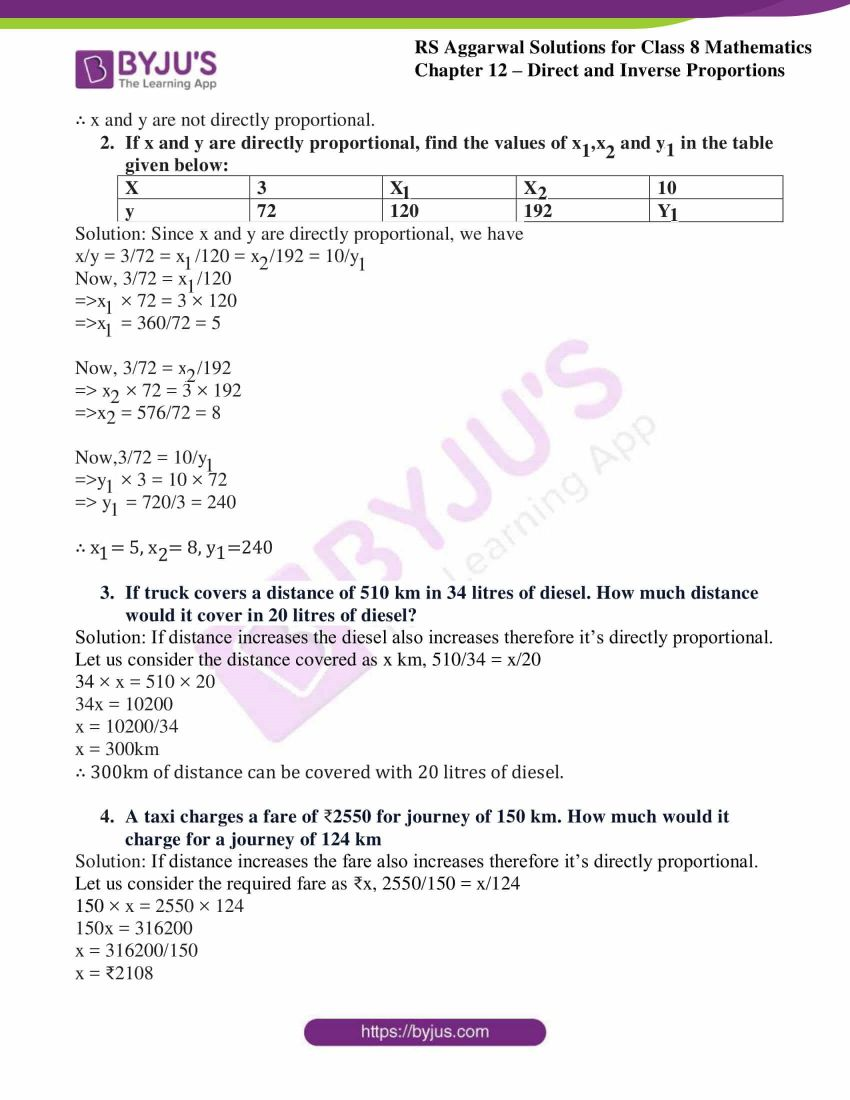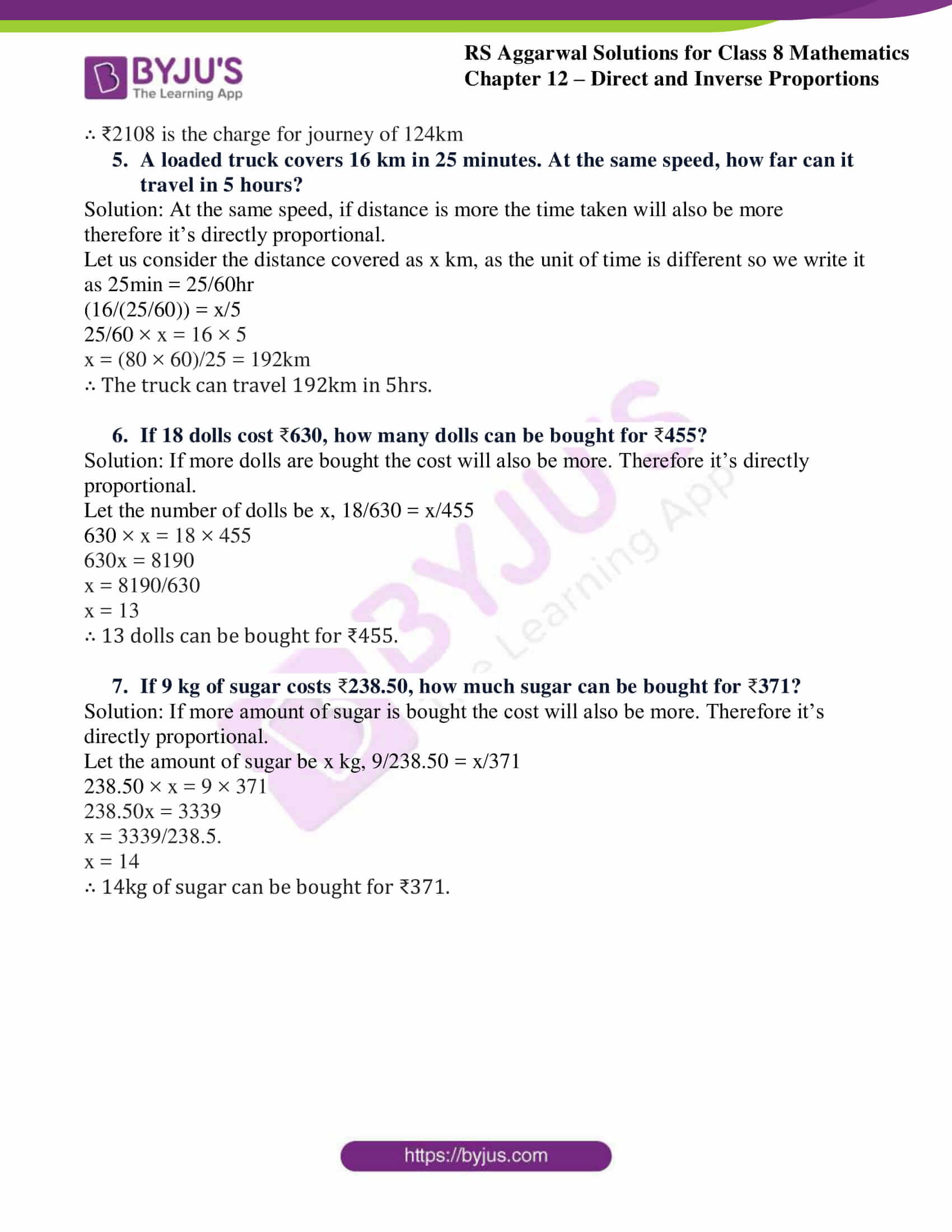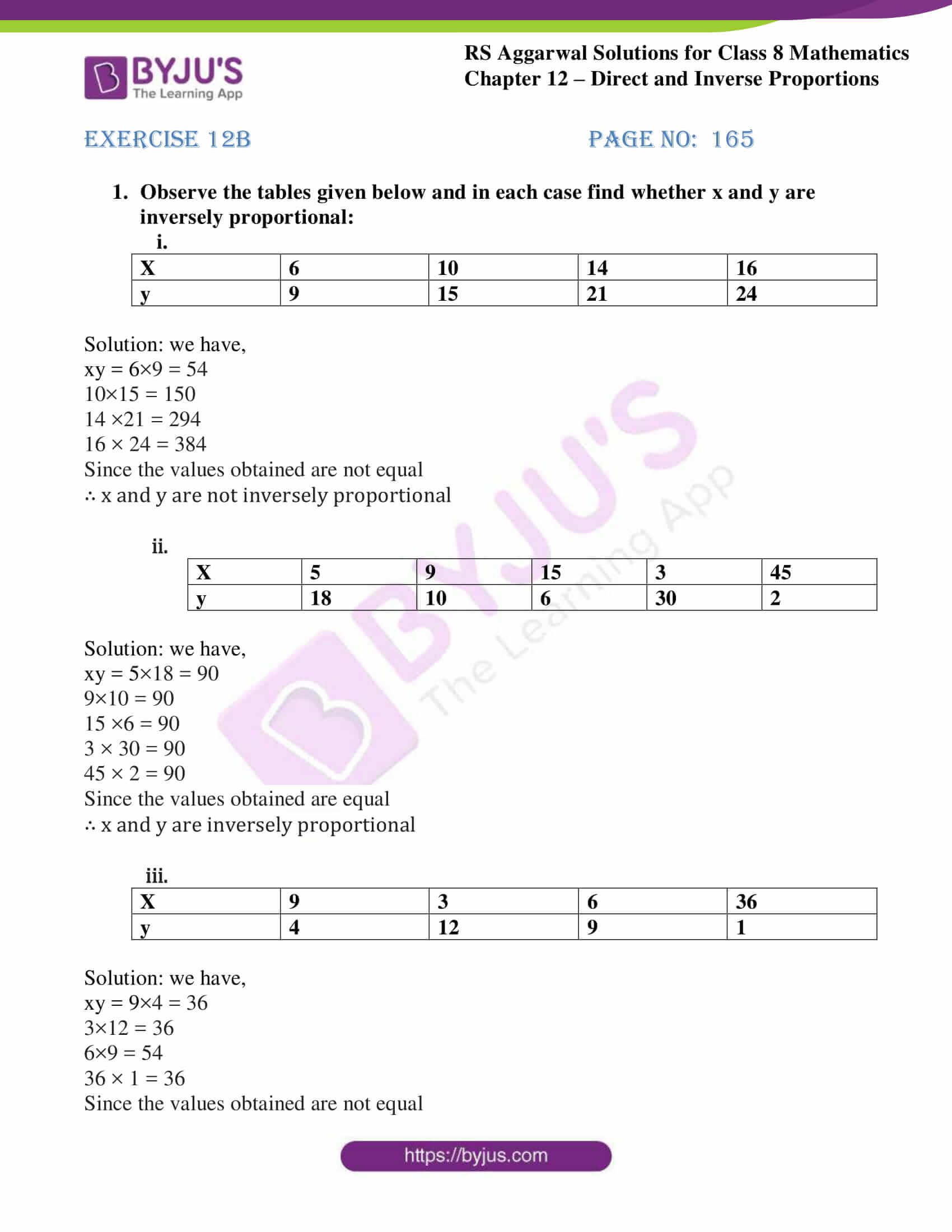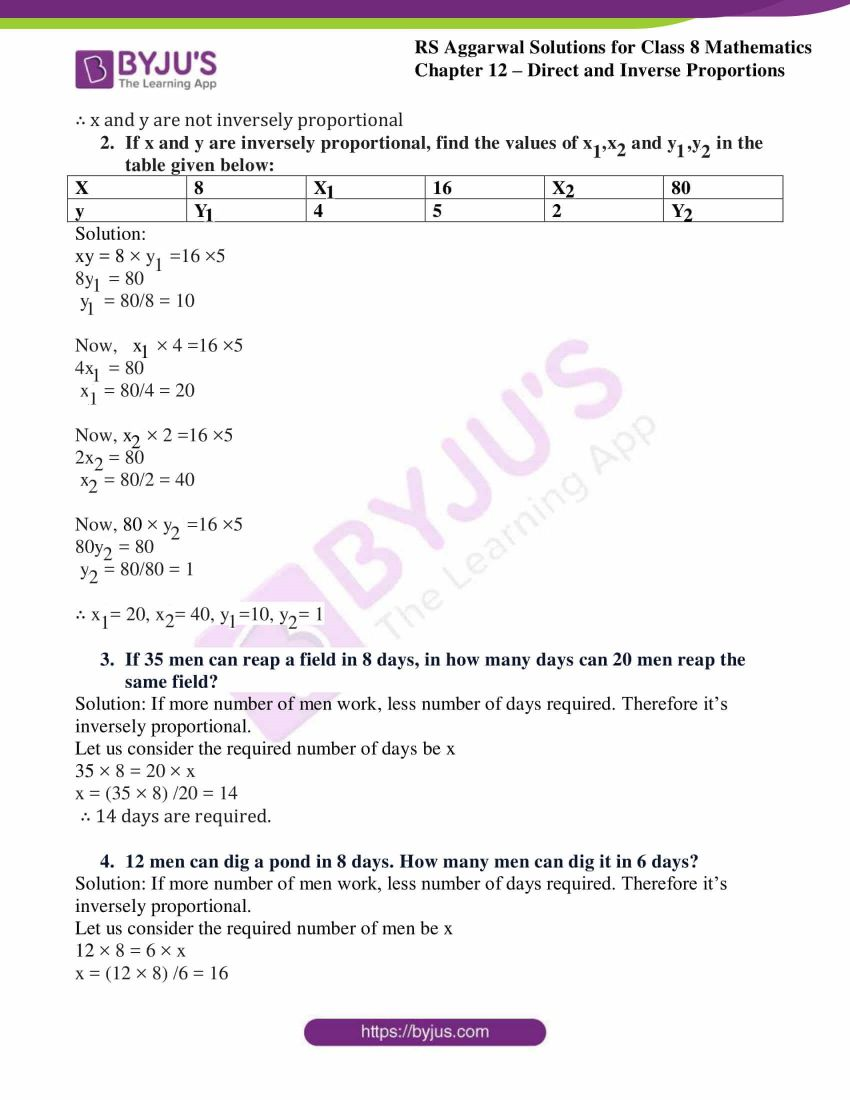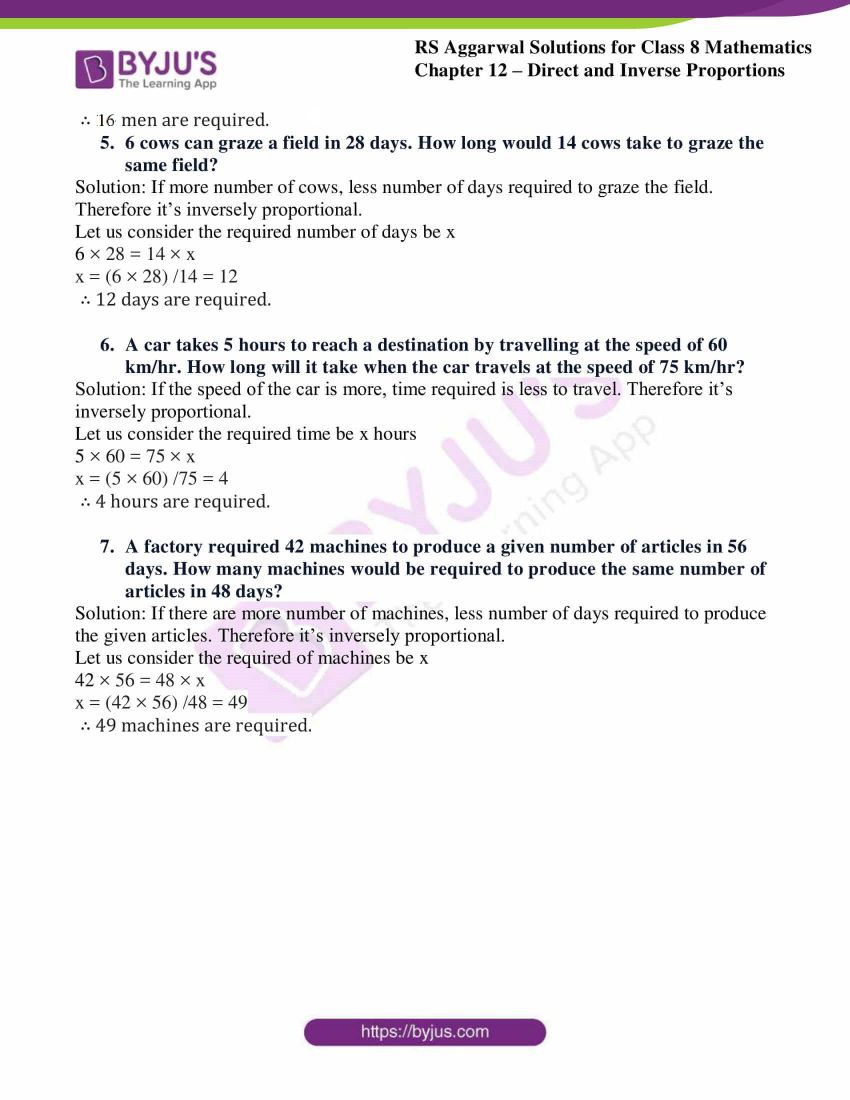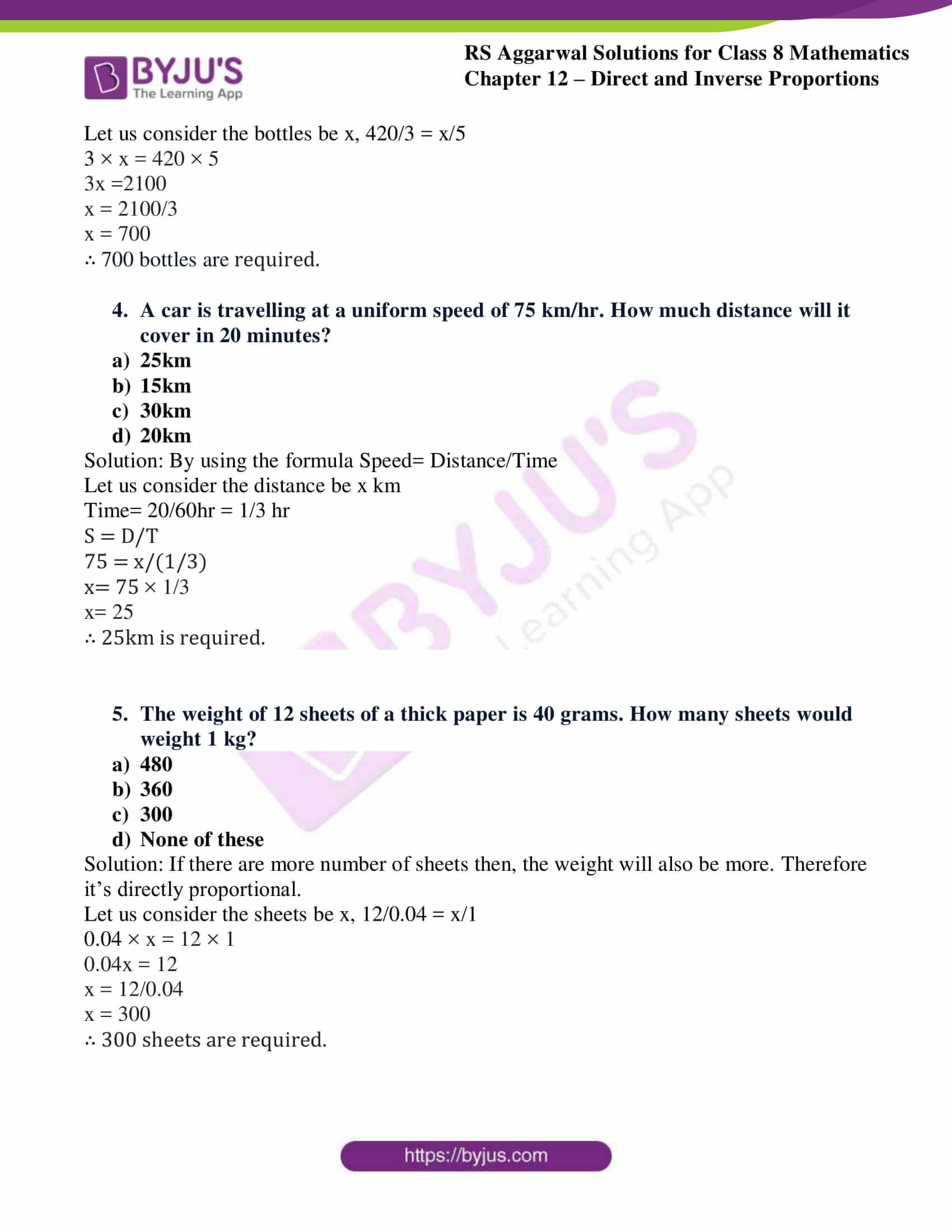## Exercise 12A

1. Observe the table given below and in each one find whether x and y are proportional:
(i).

 X 3 5 8 11 26 y 9 15 24 33 78

Solution: Here we are checking the ratio i.e.

3/9 = 1/3

5/15 = 1/3

8/24 = 1/3

11/33 = 1/3

26/78 = 1/3

Above given numbers are equally divisible.

∴ x and y are directly proportional.

(ii).

 X 2.5 4 7.5 10 14 y 10 16 30 40 42

Solution: Here we are checking the ratio i.e.

2.5/10 = ¼

4/16 = ¼

7.5/30 = ¼

10/40 = ¼

14/42 = 1/3

Above given numbers are not equal.

∴ x and y are not directly proportional.

(iii).

 X 5 7 9 15 18 25 y 15 21 27 60 72 75

Solution: Here we are checking the ratio i.e.

5/15 = 1/3

7/21 = 1/3

9/27 = 1/3

15/60 = ¼

18/72 = ¼

25/5 = 1/3

Above given numbers are not equal.

∴ x and y are not directly proportional.

2. If x and y are directly proportional, find the values of x1,x2 and y1 in the table given below:

 x 3 x1 x2 10 y 72 120 192 y1

Solution: Since x and y are directly proportional, we have

x/y = 3/72 = x1/120 = x2/192 = 10/y1

Now, 3/72 = x1/120

⇨ x1 × 72 = 3 × 120

⇨ x1 = 360/72 = 5

Now, 3/72 = x2/192

⇨ x2 × 72 = 3 × 192

⇨ x2 = 576/72 = 8

Now,3/72 = 10/y1

⇨ y1 × 3 = 10 × 72

⇨ y1 = 720/3 = 240

∴ x1= 5, x2= 8, y1=240

3. If truck covers a distance of 510 km in 34 litres of diesel. How much distance would it cover in 20 litres of diesel?

Solution: If distance increases the diesel also increases therefore it’s directly proportional.

Let us consider the distance covered as x km, 510/34 = x/20

34 × x = 510 × 20

34x = 10200

x = 10200/34

x = 300km

∴ 300km of distance can be covered with 20 litres of diesel.

4. A taxi charges a fare of 2550 for journey of 150 km. How much would it charge for a journey of 124 km

Solution: If distance increases the fare also increases therefore it’s directly proportional.

Let us consider the required fare as ₹x, 2550/150 = x/124

150 × x = 2550 × 124

150x = 316200

x = 316200/150

x = ₹2108

∴ ₹2108 is the charge for journey of 124km

5. A loaded truck covers 16 km in 25 minutes. At the same speed, how far can it travel in 5 hours?

Solution: At the same speed, if distance is more the time taken will also be more therefore it’s directly proportional.

Let us consider the distance covered as x km, as the unit of time is different so we write it as 25min = 25/60hr

(16/(25/60)) = x/5

25/60 × x = 16 × 5

x = (80 × 60)/25 = 192km

∴ The truck can travel 192km in 5hrs.

6. If 18 dolls cost 630, how many dolls can be bought for 455?

Solution: If more dolls are bought the cost will also be more. Therefore it’s directly proportional.

Let the number of dolls be x, 18/630 = x/455

630 × x = 18 × 455

630x = 8190

x = 8190/630

x = 13

∴ 13 dolls can be bought for ₹455.

7. If 9 kg of sugar costs 238.50, how much sugar can be bought for 371?

Solution: If more amount of sugar is bought the cost will also be more. Therefore it’s directly proportional.

Let the amount of sugar be x kg, 9/238.50 = x/371

238.50 × x = 9 × 371

238.50x = 3339

x = 3339/238.5.

x = 14

∴ 14kg of sugar can be bought for ₹371.

## Exercise 12B

1. Observe the tables given below and in each case find whether x and y are inversely proportional:
(i).

 X 6 10 14 16 y 9 15 21 24

Solution: we have,

xy = 6×9 = 54

10×15 = 150

14 ×21 = 294

16 × 24 = 384

Since the values obtained are not equal

∴ x and y are not inversely proportional

(ii).

 X 5 9 15 3 45 y 18 10 6 30 2

Solution: we have,

xy = 5×18 = 90

9×10 = 90

15 ×6 = 90

3 × 30 = 90

45 × 2 = 90

Since the values obtained are equal

∴ x and y are inversely proportional

(iii).

 X 9 3 6 36 y 4 12 9 1

Solution: we have,

xy = 9×4 = 36

3×12 = 36

6×9 = 54

36 × 1 = 36

Since the values obtained are not equal

∴ x and y are not inversely proportional

2. If x and y are inversely proportional, find the values of x1,x2 and y1,y2 in the table given below:

 x 8 x1 16 x2 80 y y1 4 5 2 y2

Solution:

xy = 8 × y1 =16 ×5

8y1 = 80

y1 = 80/8 = 10

Now, x1 × 4 =16 ×5

4x1 = 80

x1 = 80/4 = 20

Now, x2 × 2 =16 ×5

2x2 = 80

x2 = 80/2 = 40

Now, 80 × y2 =16 ×5

80y2 = 80

y2 = 80/80 = 1

∴ x1= 20, x2= 40, y1=10, y2= 1

3. If 35 men can reap a field in 8 days, in how many days can 20 men reap the same field?

Solution: If more number of men work, less number of days required. Therefore it’s inversely proportional.

Let us consider the required number of days be x

35 × 8 = 20 × x

x = (35 × 8) /20 = 14

∴ 14 days are required.

4. 12 men can dig a pond in 8 days. How many men can dig it in 6 days?

Solution: If more number of men work, less number of days required. Therefore it’s inversely proportional.

Let us consider the required number of men be x

12 × 8 = 6 × x

x = (12 × 8) /6 = 16

∴ 16 men are required.

5. 6 cows can graze a field in 28 days. How long would 14 cows take to graze the same field?

Solution: If more number of cows, less number of days required to graze the field. Therefore it’s inversely proportional.

Let us consider the required number of days be x

6 × 28 = 14 × x

x = (6 × 28) /14 = 12

∴ 12 days are required.

6. A car takes 5 hours to reach a destination by travelling at the speed of 60 km/hr. How long will it take when the car travels at the speed of 75 km/hr?

Solution: If the speed of the car is more, time required is less to travel. Therefore it’s inversely proportional.

Let us consider the required time be x hours

5 × 60 = 75 × x

x = (5 × 60) /75 = 4

∴ 4 hours are required.

7. A factory required 42 machines to produce a given number of articles in 56 days. How many machines would be required to produce the same number of articles in 48 days?

Solution: If there are more number of machines, less number of days required to produce the given articles. Therefore it’s inversely proportional.

Let us consider the required of machines be x

42 × 56 = 48 × x

x = (42 × 56) /48 = 49

∴ 49 machines are required.

## Exercise 12C

Select the correct answer in each of the following:

1. If 14 kg of pulses cost ₹882, what is the cost of 22 kg of pulses?

1. ₹1254
2. ₹1298
3. ₹1342
4. ₹1386

Solution: If more amount of pulses, the cost will be more. Therefore it’s directly proportional.

Let us consider the cost be x, 882/14 = x/22

14 × x = 882 × 22

14x = 19404

x = 19404/14

x = 1386

∴ ₹1386 is the cost

2. If 8 orange cost ₹52, how many oranges can be bought for ₹169?

1. 13
2. 18
3. 26
4. 24

Solution: If more amount of oranges, the cost will be more. Therefore it’s directly proportional.

Let us consider the amount be x, 8/52 = x/169

52 × x = 8 × 169

52x = 1352

x = 1352/52

x = 26

∴ 26 oranges can be bought.

3. A machine fills 420 bottles in 3 hours. How many bottles will it fill in 5 hours?

1. 252
2. 700
3. 504
4. 300

Solution: If more number of
bottles required then, time taken will also be more. Therefore it’s directly proportional.

Let us consider the bottles be x, 420/3 = x/5

3 × x = 420 × 5

3x =2100

x = 2100/3

x = 700

∴ 700 bottles are required.

4. A car is travelling at a uniform speed of 75 km/hr. How much distance will it cover in 20 minutes?

1. 25km
2. 15km
3. 30km
4. 20km

Solution: By using the formula Speed= Distance/Time

Let us consider the distance be x km

Time= 20/60hr = 1/3 hr

S = D/T

75 = x/(1/3)

x= 75 × 1/3

x= 25

∴ 25km is required.

5. The weight of 12 sheets of a thick paper is 40 grams. How many sheets would weight 1 kg?

1. 480
2. 360
3. 300
4. None of these

Solution: If there are more number of sheets then, the weight will also be more. Therefore it’s directly proportional.

Let us consider the sheets be x, 12/0.04 = x/1

0.04 × x = 12 × 1

0.04x = 12

x = 12/0.04

x = 300

∴ 300 sheets are required.

## RS Aggarwal Solutions for Class 8 Maths Chapter 12 – Direct and Inverse Proportions

Chapter 12- Direct and Inverse Proportions contain three exercises and the RS Aggarwal solutions present on this page provide the solutions for the questions present in each exercise. Now, let us have a look at the concepts discussed in this chapter.

• Variation.
• Direct Variation.
• Inverse proportion.

Exercise 12A

Exercise 12B

Exercise 12C

### Chapter Brief of RS Aggarwal Solutions for Class 8 Maths Chapter 12 – Direct and Inverse Proportions

RS Aggarwal Solutions for Class 8 Maths Chapter 12 – Direct and Inverse Proportions, ensures that the students are thorough and familiar with the concepts. Regular revision of important concepts and formulas over time to time is the best way to strengthen your concepts.

As the Chapter is about Direct and Inverse Proportions, Here are some instances where we come across, more items we buy, more the cost is; more the distance covered by a car, more the petrol consumed; more pipes will fill the tank in less time; fewer men take more days to finish the work. So here in RS Aggarwal Solutions book, many such exercise problems are given, which enhances familiarity with the concepts.